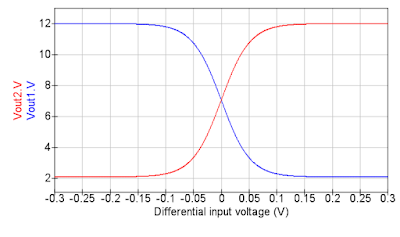## Oct 20, 2015

### Discrete VCA

This is a very simple Voltage controlled amplifier (VCA) module, using a classic differential amplifier. The design comes from Yusynth, and I implemented it without significant changes. However, it was a good example to learn about transistor amplifiers, and I write down what I learned below.

### Long-tailed pair

Below is a schematic of a basic long-tailed pair differential amplifier, which is the heart of the VCA circuit. A good complete description can be found on the Analog Devices Wiki, but here I try to give a basic understanding of the function.Basic differential amplifier

The amplifier consists of 2 identical transistors, Q1 and Q2, with their emitters connected together and their collectors connected to the positive supply via resistors R1 and R2, which we also assume to be identical. The common base connection is fed by a current source which forces a total current I through the amplifier. Being a differential amplifier, it (ideally) doesn't care about the actual input voltages Vin1 and Vin2, but only about their difference vin = Vin2 - Vin1. We are also only interested in the differential output voltage, vout = Vout2 - Vout1.

First consider the case when vin = 0 V, so Vin1 = Vin2. The circuit is completely symmetric, so half of the current I goes through Q1 and half through Q2. The currents through R1 and R2 are equal, and thus Vout2 = Vout1 and vout = 0 V.

Now if we increase Vin1, transistor Q1 is turned on a bit more, so the current can more easily flow through Q1 than through Q2. As a result, more current flows through R1, so Vout1 goes down (at the same time, less current flows through R2 and Vout2 goes up). This makes vout now negative, so our amplifier is inverting.

One is usually interested in the small-signal gain, which is the gain for a small values of vin. It can be calculated from the transistor equation I wrote in the transistor matching post using linear approximation, but here I only give the result (see also the Analog Devices article for a calculation, but it skips the first steps):

where R = R1 = R2, is the current through the current source and VT is the thermal voltage, 25 mV at room temperature. The important feature is that the gain scales with I, so we can make a VCA if we have a voltage controlled current source.

The small-signal gain equation is valid when vin is much smaller than 2VT. For larger input signals the amplifier saturates. Here is a simulation of the output voltages as function of vin. Note how Vout1 and Vout2 are each others opposite, reflecting the symmetry of the circuit. The linear input range is a few 10s of millivolts, as expected. The simulated small-signal gain is 190, close to the expected value of 200.Simulated long-tailed pair with R1 = R2 = 10kOhm and I = 1 mA.

### Complete VCA

On to the actual VCA circuit, which is shown below. I built it as extra feature onto a Moog VCF module (which will be covered in a future blog post), using two spare opamps on a TL074. For a single VCA a TL072 could be used in stead.Complete VCA circuit

To keep the amplifier in its linear range, the input signal is divided by a facor 220 by R21 and R22. This was chosen (by ear) to amplify a +/-5V signal without much distortion. The output of the long-tailed pair is processed by an opamp differential amplifier (U1b), which turns the differential output voltage in a normal single output. It also has a gain of 22 to restore the signal to its original level.

To control the gain, a simple resistor (R3) is used as a current source. Current (and thus gain) is set by the voltage at the emitter of Q3. Q3 itself is set up as a voltage follower; the emitter voltage is always about 0.6 V below the base voltage, which is in turn set by the inverting summing amplifier U1a, which combines a CV input and potentiometer setting. If I calculated correctly, the complete circuit has a gain of 1 with about 2 V of CV input. The trimmer potentiometer R18 should be adjusted to make the output just silent when the gain is turned down.

For best results (best power supply noise rejection), Q1 and Q2 should be a matched pair. The trimmer R14 can be used to get rid of any DC offset voltage, making transistor matching a bit less critical.

Compared to Yusynth's original, I left out the input summing amplifier, input level pot and LED. Some resistor values are changed - notably I reduced the signal level in the differential pair (changed R21, R6 and R7). These values worked for me to process a 5V-peak signal without too much distortion, but it is worth to play with the values.